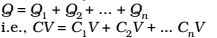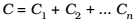## Capacitors in series:

• Fig.a shows capacitors C1 and C2 combined in series.Fig.a. Combination of two capacitors in series

• The left plate of C1 and the right plate of C2 are connected to two terminals of a battery and have charges Q and –Q, respectively.
• It then follows that the right plate ofC1 has charge –Q and the left plate of C2 has charge Q. If this was not so, the net charge on each capacitor would not be zero.
• The charge would flow until the net charge on both C1 and C2 is zero and there is no electric field in the conductor connecting C1 and C2.
• Thus, in the series combination, charges on the two plates (±Q) are the same on each capacitor.
• The total potential drop V across the combination is the sum of the potential drops V1 and V2 across C1 and C2, respectively.• The effective capacitance of the combination is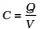So,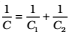• For n capacitor arranged in series (Fig. b)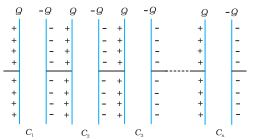Fig.b Combination of n capacitors in series

• The total potential drop V of a series combination of n capacitor is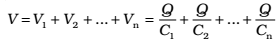• Effective capacitance of a series combination of n capacitors,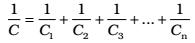## Capacitors in Parallel:

• Fig. a, shows two capacitors arranged in parallel.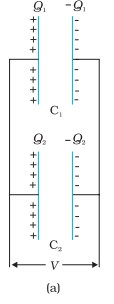Fig. Parallel combination of two capacitors

• In this case, the same potential difference is applied across both the capacitors. But the plate charges (±Q1) on capacitor1 and the plate charges (±Q2) on the capacitor 2 are not necessarily the same: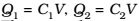• The equivalent capacitor is one with charge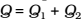and potential difference V.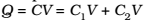• The effective capacitance C is,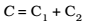• The general formula for effective capacitance C for parallel combination of n capacitors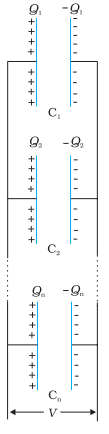Fig. Parallel combination of n capacitors